# 199 My List of Multiplication Rhymes

• 199 is a prime number.
• Prime factorization: 199 is prime.
• The exponent of prime number 199 is 1. Adding 1 to that exponent we get (1 + 1) = 2. Therefore 199 has exactly 2 factors.
• Factors of 199: 1, 199
• Factor pairs: 199 = 1 x 199
• 199 has no square factors that allow its square root to be simplified. √199 ≈ 14.1067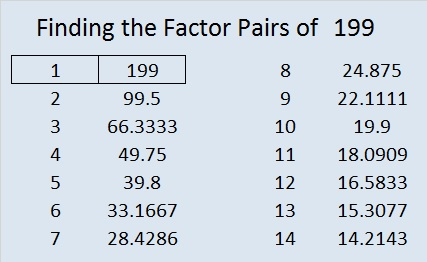How do we know that 199 is a prime number? If 199 were not a prime number, then it would be divisible by at least one prime number less than or equal to √199 ≈ 14.1. Since 199 cannot be divided evenly by 2, 3, 5, 7, 11, or 13, we know that 199 is a prime number.

———————————

I recently wrote a post that asked “Are Multiplication Rhymes Able to Help Kids Learn the Multiplication Table?” Here is a list of rhymes that I’ve put together.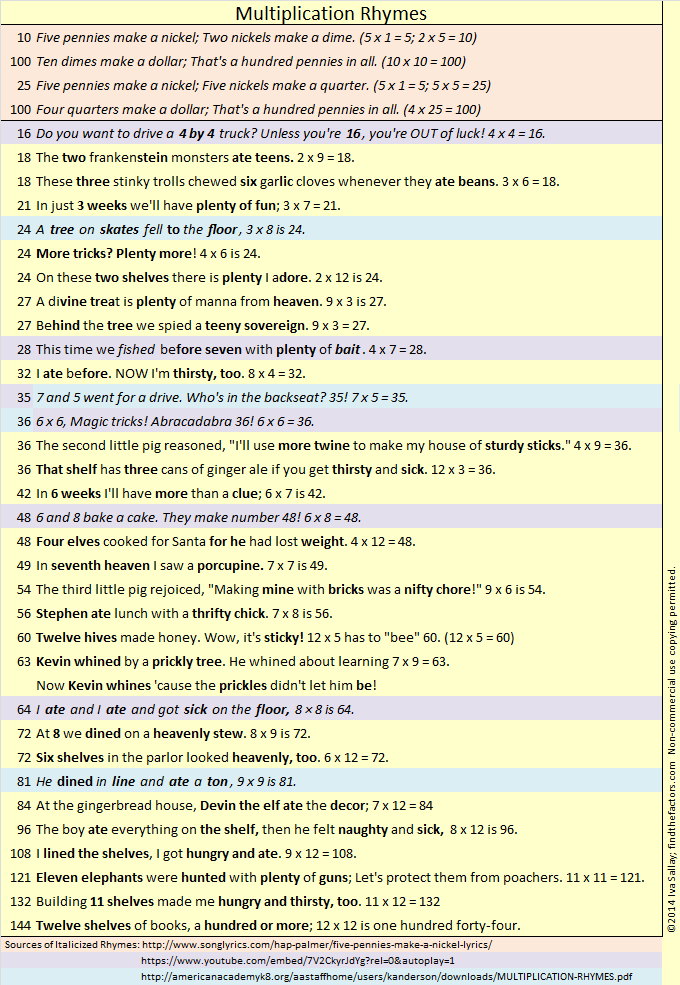Most of the rhymes have rhyming clues to help students remember both numbers being multiplied as well as their product. I wrote the rhymes that are highlighted in yellow. Those highlighted in peach, purple, or blue are from other sources, but I did alter the rhymes for 24, 28, and 81. A black and white pdf of the rhymes is also available here.

# 197 and Level 5

• 197 is a prime number.
• Prime factorization: 197 is prime.
• The exponent of prime number 197 is 1. Adding 1 to that exponent we get (1 + 1) = 2. Therefore 197 has exactly 2 factors.
• Factors of 197: 1, 197
• Factor pairs: 197 = 1 x 197
• 197 has no square factors that allow its square root to be simplified. √197 ≈ 14.0356688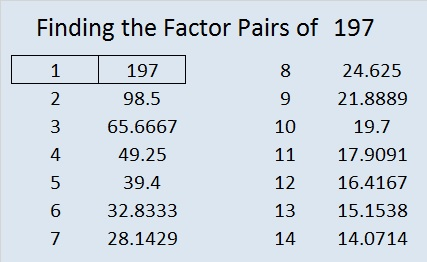How do we know that 197 is a prime number? If 197 were not a prime number, then it would be divisible by at least one prime number less than or equal to √197 ≈ 14. Since 197 cannot be divided evenly by 2, 3, 5, 7, 11, or 13, we know that 197 is a prime number.Excel file of puzzles and previous week’s factor solutions: 10 Factors 2014-08-04# 193 and Level 2

• 193 is a prime number.
• Prime factorization: 193 is prime.
• The exponent of prime number 193 is 1. Adding 1 to that exponent we get (1 + 1) = 2. Therefore 193 has exactly 2 factors.
• Factors of 193: 1, 193
• Factor pairs: 193 = 1 x 193
• 193 has no square factors that allow its square root to be simplified. √193 ≈ 13.89244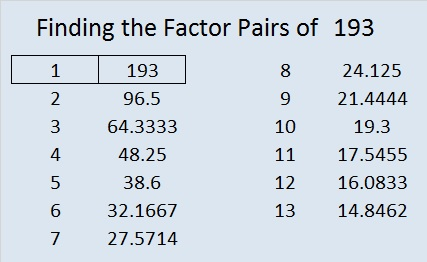How do we know that 193 is a prime number? If 193 were not a prime number, then it would be divisible by at least one prime number less than or equal to √193 ≈ 13.9. Since 193 cannot be divided evenly by 2, 3, 5, 7, 11, or 13, we know that 193 is a prime number.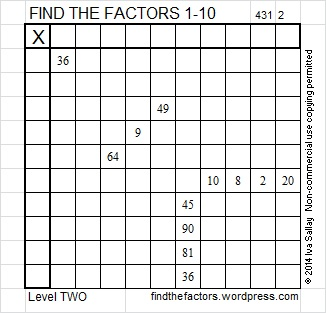Excel file of puzzles and previous week’s factor solutions: 10 Factors 2014-08-04# 191 and Level 6

• 191 is a prime number.
• Prime factorization: 191 is prime.
• The exponent of prime number 191 is 1. Adding 1 to that exponent we get (1 + 1) = 2. Therefore 191 has exactly 2 factors.
• Factors of 191: 1, 191
• Factor pairs: 191 = 1 x 191
• 191 has no square factors that allow its square root to be simplified. √191 ≈ 13.82027How do we know that 191 is a prime number? If 191 were not a prime number, then it would be divisible by at least one prime number less than or equal to √191 ≈ 13.8. Since 191 cannot be divided evenly by 2, 3, 5, 7, 11, or 13, we know that 191 is a prime number.

There are 22 prime numbers between 100 and 200:

• The first four of those prime numbers are 101, 103, 107, and 109.
• The last four of those prime numbers are 191, 193, 197, and 199.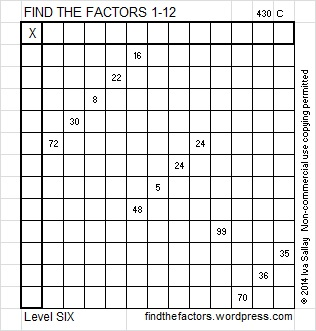Excel file of puzzles and previous week’s factor solutions: 12 Factors 2014-07-28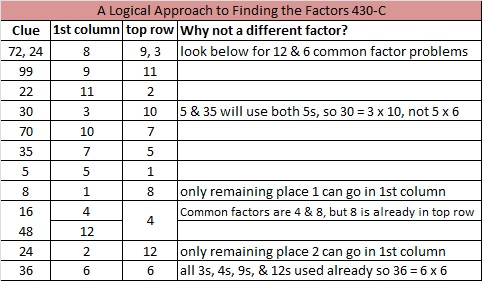# 181 and Level 2

• 181 is a prime number.
• Prime factorization: 181 is prime.
• The exponent of prime number 181 is 1. Adding 1 to that exponent we get (1 + 1) = 2. Therefore 181 has exactly 2 factors.
• Factors of 181: 1, 181
• Factor pairs: 181 = 1 x 181
• 181 has no square factors that allow its square root to be simplified. √181 ≈ 13.45362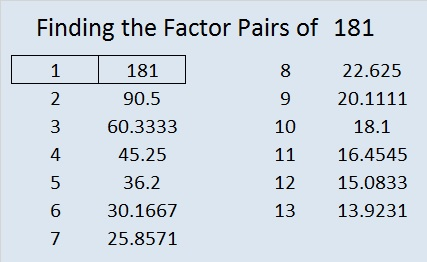How do we know that 181 is a prime number? If 181 were not a prime number, then it would be divisible by at least one prime number less than or equal to √181 ≈ 13.5. Since 181 cannot be divided evenly by 2, 3, 5, 7, 11, or 13, we know that 181 is a prime number.Excel file of puzzles and previous week’s factor solutions: 10 Factors 2014-07-21# 179 and Level 6

• 179 is a prime number.
• Prime factorization: 179 is prime.
• The exponent of prime number 179 is 1. Adding 1 to that exponent we get (1 + 1) = 2. Therefore 179 has exactly 2 factors.
• Factors of 179: 1, 179
• Factor pairs: 179 = 1 x 179
• 179 has no square factors that allow its square root to be simplified. √179 ≈ 13.379088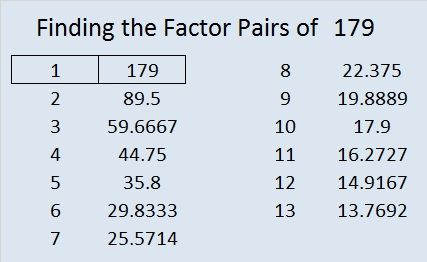How do we know that 179 is a prime number? If 179 were not a prime number, then it would be divisible by at least one prime number less than or equal to √179 ≈ 13.4. Since 179 cannot be divided evenly by 2, 3, 5, 7, 11, or 13, we know that 179 is a prime number.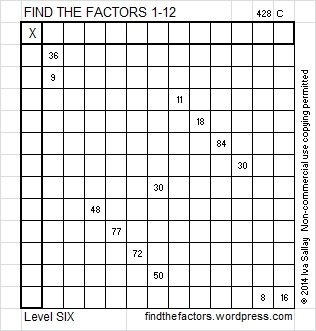Excel file of puzzles and previous week’s factor solutions: 12 Factors 2014-07-14